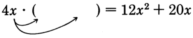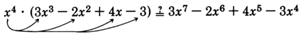# 6.2 Factoring a monomial from a polynomial

 Page 1 / 1
This module is from Elementary Algebra by Denny Burzynski and Wade Ellis, Jr. Factoring is an essential skill for success in algebra and higher level mathematics courses. Therefore, we have taken great care in developing the student's understanding of the factorization process. The technique is consistently illustrated by displaying an empty set of parentheses and describing the thought process used to discover the terms that are to be placed inside the parentheses.The factoring scheme for special products is presented with both verbal and symbolic descriptions, since not all students can interpret symbolic descriptions alone. Two techniques, the standard "trial and error" method, and the "collect and discard" method (a method similar to the "ac" method), are presented for factoring trinomials with leading coefficients different from 1. Objectives of this module: be able to factor a monomial from a polynomial.

## Overview

• The Factorization Process

## The factorization process

We introduce the process of factoring a monomial from a polynomial by examining a problem: Suppose that $12{x}^{2}+20x$ is the product and one of the factors is $4x$ . To find the other factor we could set up the problem this way:

$4x\cdot \left(\text{\hspace{0.17em}}\text{\hspace{0.17em}}\text{\hspace{0.17em}}\text{\hspace{0.17em}}\text{\hspace{0.17em}}\text{\hspace{0.17em}}\text{\hspace{0.17em}}\text{\hspace{0.17em}}\text{\hspace{0.17em}}\text{\hspace{0.17em}}\text{\hspace{0.17em}}\text{\hspace{0.17em}}\text{\hspace{0.17em}}\text{\hspace{0.17em}}\text{\hspace{0.17em}}\right)=12{x}^{2}+20x$

Since the product $12{x}^{2}+20x$ consists of two terms, the expression multiplying $4x$ must consist of two terms, since, by the distributive propertyNow we see that this problem is simply an extension of finding the factors of a monomial.

$\begin{array}{rrrrrrrrr}\hfill 1st\text{\hspace{0.17em}}term:& \hfill 4x\left(\text{\hspace{0.17em}}\text{\hspace{0.17em}}\text{\hspace{0.17em}}\text{\hspace{0.17em}}\text{\hspace{0.17em}}\text{\hspace{0.17em}}\text{\hspace{0.17em}}\text{\hspace{0.17em}}\text{\hspace{0.17em}}\text{\hspace{0.17em}}\text{\hspace{0.17em}}\text{\hspace{0.17em}}\text{\hspace{0.17em}}\text{\hspace{0.17em}}\text{\hspace{0.17em}}\right)& \hfill =& \hfill 12{x}^{2}& \hfill & \hfill 2nd\text{\hspace{0.17em}}term:& \hfill 4x\left(\text{\hspace{0.17em}}\text{\hspace{0.17em}}\text{\hspace{0.17em}}\text{\hspace{0.17em}}\text{\hspace{0.17em}}\text{\hspace{0.17em}}\text{\hspace{0.17em}}\text{\hspace{0.17em}}\text{\hspace{0.17em}}\text{\hspace{0.17em}}\text{\hspace{0.17em}}\text{\hspace{0.17em}}\text{\hspace{0.17em}}\text{\hspace{0.17em}}\text{\hspace{0.17em}}\right)& \hfill =& \hfill 20x\\ \hfill & \hfill \left(\text{\hspace{0.17em}}\text{\hspace{0.17em}}\text{\hspace{0.17em}}\text{\hspace{0.17em}}\text{\hspace{0.17em}}\text{\hspace{0.17em}}\text{\hspace{0.17em}}\text{\hspace{0.17em}}\text{\hspace{0.17em}}\text{\hspace{0.17em}}\text{\hspace{0.17em}}\text{\hspace{0.17em}}\text{\hspace{0.17em}}\text{\hspace{0.17em}}\text{\hspace{0.17em}}\right)& \hfill =& \hfill \frac{12{x}^{2}}{4x}& \hfill & \hfill & \hfill \left(\text{\hspace{0.17em}}\text{\hspace{0.17em}}\text{\hspace{0.17em}}\text{\hspace{0.17em}}\text{\hspace{0.17em}}\text{\hspace{0.17em}}\text{\hspace{0.17em}}\text{\hspace{0.17em}}\text{\hspace{0.17em}}\text{\hspace{0.17em}}\text{\hspace{0.17em}}\text{\hspace{0.17em}}\text{\hspace{0.17em}}\text{\hspace{0.17em}}\text{\hspace{0.17em}}\right)& \hfill =& \hfill \frac{20x}{4x}\\ \hfill & \hfill \left(\text{\hspace{0.17em}}\text{\hspace{0.17em}}\text{\hspace{0.17em}}\text{\hspace{0.17em}}\text{\hspace{0.17em}}\text{\hspace{0.17em}}\text{\hspace{0.17em}}\text{\hspace{0.17em}}\text{\hspace{0.17em}}\text{\hspace{0.17em}}\text{\hspace{0.17em}}\text{\hspace{0.17em}}\text{\hspace{0.17em}}\text{\hspace{0.17em}}\text{\hspace{0.17em}}\right)& \hfill =& 3x\hfill & \hfill & \hfill & \hfill \left(\text{\hspace{0.17em}}\text{\hspace{0.17em}}\text{\hspace{0.17em}}\text{\hspace{0.17em}}\text{\hspace{0.17em}}\text{\hspace{0.17em}}\text{\hspace{0.17em}}\text{\hspace{0.17em}}\text{\hspace{0.17em}}\text{\hspace{0.17em}}\text{\hspace{0.17em}}\text{\hspace{0.17em}}\text{\hspace{0.17em}}\text{\hspace{0.17em}}\text{\hspace{0.17em}}\right)& \hfill =& 5\hfill \end{array}$

Thus, $4x\cdot \left(3x+5\right)=12{x}^{2}+20x$ .

Usually, these divisions can be done mentally and the terms of the factor filled in directly.

## Sample set a

The product is $3{x}^{7}-2{x}^{6}+4{x}^{5}-3{x}^{4}$ and one factor is ${x}^{4}$ . Find the other factor.

We have the problem: ${x}^{4}$ times "what expression" yields $3{x}^{7}-2{x}^{6}+4{x}^{5}-3{x}^{4}$ ? Mathematically,

${x}^{4}\cdot \left(\text{\hspace{0.17em}}\text{\hspace{0.17em}}\text{\hspace{0.17em}}\text{\hspace{0.17em}}\text{\hspace{0.17em}}\text{\hspace{0.17em}}\text{\hspace{0.17em}}\text{\hspace{0.17em}}\text{\hspace{0.17em}}\text{\hspace{0.17em}}\text{\hspace{0.17em}}\text{\hspace{0.17em}}\text{\hspace{0.17em}}\text{\hspace{0.17em}}\text{\hspace{0.17em}}\right)=3{x}^{7}-2{x}^{6}+4{x}^{5}-3{x}^{4}$

Since there are four terms in the product, there must be four terms inside the parentheses. To find each of the four terms, we’ll divide (mentally) each term of the product by ${x}^{4}$ . The resulting quotient will be the necessary term of the factor.

$\begin{array}{lllll}1st\text{\hspace{0.17em}}term:\hfill & \frac{3{x}^{7}}{{x}^{4}}\hfill & =\hfill & 3{x}^{7-4}=3{x}^{3}\hfill & \text{Place}\text{\hspace{0.17em}}3{x}^{3}\text{\hspace{0.17em}}\text{into}\text{\hspace{0.17em}}\text{the}\text{\hspace{0.17em}}1\text{st}\text{\hspace{0.17em}}\text{position}\text{\hspace{0.17em}}\text{in}\text{\hspace{0.17em}}\text{the}\left(\begin{array}{cc}& \end{array}\right).\hfill \\ 2nd\text{\hspace{0.17em}}term:\hfill & \frac{-2{x}^{6}}{{x}^{4}}\hfill & =\hfill & -2{x}^{2}\hfill & \text{Place}\text{\hspace{0.17em}}-2{x}^{2}\text{\hspace{0.17em}}\text{into}\text{\hspace{0.17em}}\text{the}\text{\hspace{0.17em}}2\text{nd}\text{\hspace{0.17em}}\text{position}\text{\hspace{0.17em}}\text{in}\text{\hspace{0.17em}}\text{the}\left(\begin{array}{cc}& \end{array}\right).\hfill \\ 3rd\text{\hspace{0.17em}}term:\hfill & \frac{4{x}^{5}}{{x}^{4}}\hfill & =\hfill & 4x\hfill & \text{Place}\text{\hspace{0.17em}}4x\text{\hspace{0.17em}}\text{into}\text{\hspace{0.17em}}\text{the}\text{\hspace{0.17em}}3\text{rd}\text{\hspace{0.17em}}\text{position}\text{\hspace{0.17em}}\text{in}\text{\hspace{0.17em}}\text{the}\left(\begin{array}{cc}& \end{array}\right).\hfill \\ 4th\text{\hspace{0.17em}}term:\hfill & \frac{-3{x}^{4}}{{x}^{4}}\hfill & =\hfill & -3\hfill & \text{Place}\text{\hspace{0.17em}}-3\text{\hspace{0.17em}}\text{into}\text{\hspace{0.17em}}\text{the}\text{\hspace{0.17em}}4\text{th}\text{\hspace{0.17em}}\text{position}\text{\hspace{0.17em}}\text{in}\text{\hspace{0.17em}}\text{the}\left(\begin{array}{cc}& \end{array}\right).\hfill \end{array}$

Therefore, the other factor is $3{x}^{3}-2{x}^{2}+4x-3$ .

This result can be checked by applying the distributive property.

$\begin{array}{rrrr}\hfill {x}^{4}\cdot \left(3{x}^{3}-2{x}^{2}+4x-3\right)& \hfill =& \hfill 3{x}^{7}-2{x}^{6}+4{x}^{5}-3{x}^{4}& \hfill \text{Is this correct?}\end{array}$$\begin{array}{l}\begin{array}{rrrr}\hfill 3{x}^{4+3}-2{x}^{4+2}+4{x}^{4+1}& \hfill =& \hfill 3{x}^{7}-2{x}^{6}+4{x}^{5}-3{x}^{4}& \hfill \text{Is this correct?}\end{array}\\ \begin{array}{rrrr}\hfill 3{x}^{7}-2{x}^{6}+4{x}^{5}-3{x}^{4}& \hfill =& \hfill 3{x}^{7}-2{x}^{6}+4{x}^{5}-3{x}^{4}& \hfill \text{Yes, this is correct}\text{.}\end{array}\end{array}$

Thus,

${x}^{4}\cdot \left(3{x}^{3}-2{x}^{2}+4x-3\right)=3{x}^{7}-2{x}^{6}+4{x}^{5}-3{x}^{4}$

Again, if the divisions can be performed mentally, the process can proceed very quickly.

The product is $10{x}^{3}{y}^{6}+15{x}^{3}{y}^{4}-5{x}^{2}{y}^{4}$ and a factor is $5{x}^{2}{y}^{4}$ . Find the other factor.

$5{x}^{2}{y}^{4}\cdot \left(\text{\hspace{0.17em}}\text{\hspace{0.17em}}\text{\hspace{0.17em}}\text{\hspace{0.17em}}\text{\hspace{0.17em}}\text{\hspace{0.17em}}\text{\hspace{0.17em}}\text{\hspace{0.17em}}\text{\hspace{0.17em}}\text{\hspace{0.17em}}\text{\hspace{0.17em}}\text{\hspace{0.17em}}\text{\hspace{0.17em}}\text{\hspace{0.17em}}\text{\hspace{0.17em}}\right)=10{x}^{3}{y}^{6}+15{x}^{3}{y}^{4}-5{x}^{2}{y}^{4}$

Since there are three terms in the product, there must be three terms inside the parentheses. To find each of these three terms, we’ll divide each term of the product by $5{x}^{2}{y}^{4}$ .

$\begin{array}{lllll}1st\text{\hspace{0.17em}}term:\hfill & \frac{10{x}^{3}{y}^{6}}{5{x}^{2}{y}^{4}}\hfill & =\hfill & 2x{y}^{2}\hfill & \text{Place}\text{\hspace{0.17em}}\text{the}\text{\hspace{0.17em}}2x{y}^{2}\text{\hspace{0.17em}}\text{into}\text{\hspace{0.17em}}\text{the}\text{\hspace{0.17em}}\text{1st}\text{\hspace{0.17em}}\text{position}\text{\hspace{0.17em}}\text{in}\text{\hspace{0.17em}}\text{the}\left(\begin{array}{cc}& \end{array}\right).\hfill \\ 2nd\text{\hspace{0.17em}}term:\hfill & \frac{15{x}^{3}{y}^{4}}{5{x}^{2}{y}^{4}}\hfill & =\hfill & 3x\hfill & \text{Place}\text{\hspace{0.17em}}\text{the}\text{\hspace{0.17em}}3x\text{\hspace{0.17em}}\text{into}\text{\hspace{0.17em}}\text{the}\text{\hspace{0.17em}}\text{2nd}\text{\hspace{0.17em}}\text{position}\text{\hspace{0.17em}}\text{in}\text{\hspace{0.17em}}\text{the}\left(\begin{array}{cc}& \end{array}\right).\hfill \\ 3rd\text{\hspace{0.17em}}term:\hfill & \frac{-5{x}^{2}{y}^{4}}{5{x}^{2}{y}^{4}}\hfill & =\hfill & -1\hfill & \text{Place}\text{\hspace{0.17em}}\text{the}\text{\hspace{0.17em}}-1\text{\hspace{0.17em}}\text{into}\text{\hspace{0.17em}}\text{the}\text{\hspace{0.17em}}\text{3rd}\text{\hspace{0.17em}}\text{position}\text{\hspace{0.17em}}\text{in}\text{\hspace{0.17em}}\text{the}\left(\begin{array}{cc}& \end{array}\right).\hfill \end{array}$

The other factor is $2x{y}^{2}+3x-1$ , and

$5{x}^{2}{y}^{4}\cdot \left(2x{y}^{2}+3x-1\right)=10{x}^{3}{y}^{6}+15{x}^{3}{y}^{4}-5{x}^{2}{y}^{4}$

The product is $-4{a}^{2}-{b}^{3}+2c$ and a factor is $-1$ . Find the other factor.

$-1\left(\text{\hspace{0.17em}}\text{\hspace{0.17em}}\text{\hspace{0.17em}}\text{\hspace{0.17em}}\text{\hspace{0.17em}}\text{\hspace{0.17em}}\text{\hspace{0.17em}}\text{\hspace{0.17em}}\text{\hspace{0.17em}}\text{\hspace{0.17em}}\text{\hspace{0.17em}}\text{\hspace{0.17em}}\text{\hspace{0.17em}}\text{\hspace{0.17em}}\text{\hspace{0.17em}}\right)=-4{a}^{2}-{b}^{3}+2c$

Since there are three terms in the product, there must be three terms inside the parentheses. We will divide (mentally) each term of the product by $-1$ .

$\begin{array}{lllll}1st\text{\hspace{0.17em}}term:\hfill & \frac{-4{a}^{2}}{-1}\hfill & =\hfill & 4{a}^{2}\hfill & \text{Place}\text{\hspace{0.17em}}4{a}^{2}\text{\hspace{0.17em}}\text{into}\text{\hspace{0.17em}}\text{the}\text{\hspace{0.17em}}\text{1st}\text{\hspace{0.17em}}\text{position}\text{\hspace{0.17em}}\text{inside}\text{\hspace{0.17em}}\text{the}\left(\begin{array}{cc}& \end{array}\right).\hfill \\ 2nd\text{\hspace{0.17em}}term:\hfill & \frac{-{b}^{3}}{-1}\hfill & =\hfill & {b}^{3}\hfill & \text{Place}\text{\hspace{0.17em}}{b}^{3}\text{\hspace{0.17em}}\text{into}\text{\hspace{0.17em}}\text{the}\text{\hspace{0.17em}}\text{2nd}\text{\hspace{0.17em}}\text{position}\text{\hspace{0.17em}}\text{inside}\text{\hspace{0.17em}}\text{the}\left(\begin{array}{cc}& \end{array}\right).\hfill \\ 3rd\text{\hspace{0.17em}}term:\hfill & \frac{2c}{-1}\hfill & =\hfill & -2c\hfill & \text{Place}\text{\hspace{0.17em}}-2c\text{\hspace{0.17em}}\text{into}\text{\hspace{0.17em}}\text{the}\text{\hspace{0.17em}}\text{3rd}\text{\hspace{0.17em}}\text{position}\text{\hspace{0.17em}}\text{inside}\text{\hspace{0.17em}}\text{the}\left(\begin{array}{cc}& \end{array}\right).\hfill \end{array}$

The other factor is $4{a}^{2}+{b}^{3}-2c$ , and

$-1\left(4{a}^{2}+{b}^{3}-2c\right)=-4{a}^{2}-{b}^{3}+2c$

Without writing the $-1$ , we get

$-\left(4{a}^{2}+{b}^{3}-2c\right)=-4{a}^{2}-{b}^{3}+2c$

The product is $-3{a}^{2}{b}^{5}-15{a}^{3}{b}^{2}+9{a}^{2}{b}^{2}$ and a factor is $-3{a}^{2}{b}^{2}$ . Find the other factor.

$-3{a}^{2}{b}^{2}\left(\text{\hspace{0.17em}}\text{\hspace{0.17em}}\text{\hspace{0.17em}}\text{\hspace{0.17em}}\text{\hspace{0.17em}}\text{\hspace{0.17em}}\text{\hspace{0.17em}}\text{\hspace{0.17em}}\text{\hspace{0.17em}}\text{\hspace{0.17em}}\text{\hspace{0.17em}}\text{\hspace{0.17em}}\text{\hspace{0.17em}}\text{\hspace{0.17em}}\text{\hspace{0.17em}}\right)=-3{a}^{2}{b}^{5}-15{a}^{3}{b}^{2}+9{a}^{2}{b}^{2}$

Mentally dividing each term of the original trinomial by $-3{a}^{2}{b}^{2}$ , we get ${b}^{3}+5a-3$ as the other factor, and

$-3{a}^{2}{b}^{2}\left({b}^{3}+5a-3\right)=-3{a}^{2}{b}^{5}-15{a}^{3}{b}^{2}+9{a}^{2}{b}^{2}$

## Practice set a

The product is $3{x}^{2}-6x$ and a factor is $3x$ . Find the other factor.

$x-2$

The product is $5{y}^{4}+10{y}^{3}-15{y}^{2}$ and a factor is $5{y}^{2}$ . Find the other factor.

${y}^{2}+2y-3$

The product is $4{x}^{5}{y}^{3}-8{x}^{4}{y}^{4}+16{x}^{3}{y}^{5}+24x{y}^{7}$ and a factor is $4x{y}^{3}$ . Find the other factor.

${x}^{4}-2{x}^{3}y+4{x}^{2}{y}^{2}+6{y}^{4}$

The product is $-25a^{4}-35a^{2}+5$ and a factor is $-5$ . Find the other factor.

$5{a}^{4}+7{a}^{2}-1$

The product is $-{a}^{2}+{b}^{2}$ and a factor is $-1$ . Find the other factor.

${a}^{2}-{b}^{2}$

## Exercises

For the following problems, the first quantity represents the product and the second quantity a factor. Find the other factor.

$4x+10,\text{\hspace{0.17em}}\text{\hspace{0.17em}}\text{\hspace{0.17em}}\text{\hspace{0.17em}}\text{\hspace{0.17em}}2$

$2x+5$

$6y+18,\text{\hspace{0.17em}}\text{\hspace{0.17em}}\text{\hspace{0.17em}}\text{\hspace{0.17em}}\text{\hspace{0.17em}}3$

$5x+25,\text{\hspace{0.17em}}\text{\hspace{0.17em}}\text{\hspace{0.17em}}\text{\hspace{0.17em}}\text{\hspace{0.17em}}5$

$x+5$

$16a+64,\text{\hspace{0.17em}}\text{\hspace{0.17em}}\text{\hspace{0.17em}}\text{\hspace{0.17em}}\text{\hspace{0.17em}}8$

$3{a}^{2}+9a,\text{\hspace{0.17em}}\text{\hspace{0.17em}}\text{\hspace{0.17em}}\text{\hspace{0.17em}}\text{\hspace{0.17em}}3a$

$a+3$

$14{b}^{2}+16b,\text{\hspace{0.17em}}\text{\hspace{0.17em}}\text{\hspace{0.17em}}\text{\hspace{0.17em}}\text{\hspace{0.17em}}2b$

$21{x}^{2}+28x,\text{\hspace{0.17em}}\text{\hspace{0.17em}}\text{\hspace{0.17em}}\text{\hspace{0.17em}}\text{\hspace{0.17em}}7x$

$3x+4$

$45{y}^{2}+50y,\text{\hspace{0.17em}}\text{\hspace{0.17em}}\text{\hspace{0.17em}}\text{\hspace{0.17em}}\text{\hspace{0.17em}}5y$

$18{a}^{2}-4a,\text{\hspace{0.17em}}\text{\hspace{0.17em}}\text{\hspace{0.17em}}\text{\hspace{0.17em}}\text{\hspace{0.17em}}2a$

$9a-2$

$20{a}^{2}-12a,\text{\hspace{0.17em}}\text{\hspace{0.17em}}\text{\hspace{0.17em}}\text{\hspace{0.17em}}\text{\hspace{0.17em}}4a$

$7{x}^{2}-14x,\text{\hspace{0.17em}}\text{\hspace{0.17em}}\text{\hspace{0.17em}}\text{\hspace{0.17em}}\text{\hspace{0.17em}}7x$

$x-2$

$6{y}^{2}-24y,\text{\hspace{0.17em}}\text{\hspace{0.17em}}\text{\hspace{0.17em}}\text{\hspace{0.17em}}\text{\hspace{0.17em}}6y$

$8{a}^{2}+4a,\text{\hspace{0.17em}}\text{\hspace{0.17em}}\text{\hspace{0.17em}}\text{\hspace{0.17em}}\text{\hspace{0.17em}}4a$

$2a+1$

$26{b}^{2}+13b,\text{\hspace{0.17em}}\text{\hspace{0.17em}}\text{\hspace{0.17em}}\text{\hspace{0.17em}}\text{\hspace{0.17em}}13b$

$9{x}^{2}+6x+18,\text{\hspace{0.17em}}\text{\hspace{0.17em}}\text{\hspace{0.17em}}\text{\hspace{0.17em}}\text{\hspace{0.17em}}6$

$\frac{3}{2}{x}^{2}+x+3$

$12{b}^{2}+16b+20,\text{\hspace{0.17em}}\text{\hspace{0.17em}}\text{\hspace{0.17em}}\text{\hspace{0.17em}}\text{\hspace{0.17em}}4$

$21{x}^{2}+7x-14,\text{\hspace{0.17em}}\text{\hspace{0.17em}}\text{\hspace{0.17em}}\text{\hspace{0.17em}}\text{\hspace{0.17em}}7$

$3{x}^{2}+x-2$

$35{x}^{2}+40x-5,\text{\hspace{0.17em}}\text{\hspace{0.17em}}\text{\hspace{0.17em}}\text{\hspace{0.17em}}\text{\hspace{0.17em}}5$

$14{y}^{2}-28y+14,\text{\hspace{0.17em}}\text{\hspace{0.17em}}\text{\hspace{0.17em}}\text{\hspace{0.17em}}\text{\hspace{0.17em}}14$

${y}^{2}-2y+1$

$36{a}^{2}-16a+12,\text{\hspace{0.17em}}\text{\hspace{0.17em}}\text{\hspace{0.17em}}\text{\hspace{0.17em}}\text{\hspace{0.17em}}4$

$4{y}^{2}-10y-12,\text{\hspace{0.17em}}\text{\hspace{0.17em}}\text{\hspace{0.17em}}\text{\hspace{0.17em}}\text{\hspace{0.17em}}2$

$2{y}^{2}-5y-6$

$6{b}^{2}-6b-3,\text{\hspace{0.17em}}\text{\hspace{0.17em}}\text{\hspace{0.17em}}\text{\hspace{0.17em}}\text{\hspace{0.17em}}3$

$18{x}^{3}+20x,\text{\hspace{0.17em}}\text{\hspace{0.17em}}\text{\hspace{0.17em}}\text{\hspace{0.17em}}\text{\hspace{0.17em}}2x$

$9{x}^{2}+10$

$40{y}^{3}+24y,\text{\hspace{0.17em}}\text{\hspace{0.17em}}\text{\hspace{0.17em}}\text{\hspace{0.17em}}\text{\hspace{0.17em}}4y$

$16{x}^{3}-12{x}^{2},\text{\hspace{0.17em}}\text{\hspace{0.17em}}\text{\hspace{0.17em}}\text{\hspace{0.17em}}\text{\hspace{0.17em}}4{x}^{2}$

$4x-3$

$11{x}^{3}-11x+11,\text{\hspace{0.17em}}\text{\hspace{0.17em}}\text{\hspace{0.17em}}\text{\hspace{0.17em}}\text{\hspace{0.17em}}11$

$10{a}^{3}+12{a}^{2}+16a+8,\text{\hspace{0.17em}}\text{\hspace{0.17em}}\text{\hspace{0.17em}}\text{\hspace{0.17em}}\text{\hspace{0.17em}}2$

$5{a}^{3}+6{a}^{2}+8a+4$

$14{b}^{3}+16{b}^{2}+26b+30,\text{\hspace{0.17em}}\text{\hspace{0.17em}}\text{\hspace{0.17em}}\text{\hspace{0.17em}}\text{\hspace{0.17em}}2$

$8{a}^{3}-4{a}^{2}-12a+16,\text{\hspace{0.17em}}\text{\hspace{0.17em}}\text{\hspace{0.17em}}\text{\hspace{0.17em}}\text{\hspace{0.17em}}4$

$2{a}^{3}-{a}^{2}-3a+4$

$25{x}^{3}-30{x}^{2}+15x-10,\text{\hspace{0.17em}}\text{\hspace{0.17em}}\text{\hspace{0.17em}}\text{\hspace{0.17em}}\text{\hspace{0.17em}}5$

$4{x}^{6}+16{x}^{4}-16x,\text{\hspace{0.17em}}\text{\hspace{0.17em}}\text{\hspace{0.17em}}\text{\hspace{0.17em}}\text{\hspace{0.17em}}4x$

${x}^{5}+4{x}^{3}-4$

$9{a}^{5}+6{a}^{5}-18{a}^{4}+24{a}^{2},\text{\hspace{0.17em}}\text{\hspace{0.17em}}\text{\hspace{0.17em}}\text{\hspace{0.17em}}\text{\hspace{0.17em}}3{a}^{2}$

$10{x}^{3}-35{x}^{2},\text{\hspace{0.17em}}\text{\hspace{0.17em}}\text{\hspace{0.17em}}\text{\hspace{0.17em}}\text{\hspace{0.17em}}5{x}^{2}$

$2x-7$

$12{x}^{3}{y}^{5}+20{x}^{3}{y}^{2},\text{\hspace{0.17em}}\text{\hspace{0.17em}}\text{\hspace{0.17em}}\text{\hspace{0.17em}}\text{\hspace{0.17em}}4{x}^{3}{y}^{2}$

$10{a}^{4}{b}^{3}+4{a}^{3}{b}^{4},\text{\hspace{0.17em}}\text{\hspace{0.17em}}\text{\hspace{0.17em}}\text{\hspace{0.17em}}\text{\hspace{0.17em}}2{a}^{3}{b}^{3}$

$5a+2b$

$8{a}^{3}{b}^{6}{c}^{8}+12{a}^{2}{b}^{5}{c}^{6}-16{a}^{2}{b}^{7}{c}^{5},\text{\hspace{0.17em}}\text{\hspace{0.17em}}\text{\hspace{0.17em}}\text{\hspace{0.17em}}\text{\hspace{0.17em}}4{a}^{2}{b}^{5}{c}^{5}$

$4{x}^{5}{y}^{4}+{x}^{2}+x,\text{\hspace{0.17em}}\text{\hspace{0.17em}}\text{\hspace{0.17em}}\text{\hspace{0.17em}}\text{\hspace{0.17em}}x$

$4{x}^{4}{y}^{4}+x+1$

$14{a}^{5}{b}^{2}-3{a}^{4}{b}^{4}+7{a}^{3},\text{\hspace{0.17em}}\text{\hspace{0.17em}}\text{\hspace{0.17em}}\text{\hspace{0.17em}}\text{\hspace{0.17em}}{a}^{3}$

$64{a}^{5}{b}^{3}{c}^{11}+56{a}^{4}{b}^{4}{c}^{10}-48{a}^{3}{b}^{5}{c}^{9}-8{a}^{3}{b}^{2}{c}^{5},\text{\hspace{0.17em}}\text{\hspace{0.17em}}\text{\hspace{0.17em}}\text{\hspace{0.17em}}\text{\hspace{0.17em}}8{a}^{3}{b}^{2}{c}^{5}$

$8{a}^{2}b{c}^{6}+7a{b}^{2}{c}^{5}-6{b}^{3}{c}^{4}-1$

$3{h}^{3}{b}^{2}-2{h}^{6}{b}^{3}-9{h}^{2}b+hb,\text{\hspace{0.17em}}\text{\hspace{0.17em}}\text{\hspace{0.17em}}\text{\hspace{0.17em}}\text{\hspace{0.17em}}hb$

$5a+10,\text{\hspace{0.17em}}\text{\hspace{0.17em}}\text{\hspace{0.17em}}\text{\hspace{0.17em}}\text{\hspace{0.17em}}-5$

$-a-2$

$6b+8,\text{\hspace{0.17em}}\text{\hspace{0.17em}}\text{\hspace{0.17em}}\text{\hspace{0.17em}}\text{\hspace{0.17em}}-2$

$8{x}^{2}+12x,\text{\hspace{0.17em}}\text{\hspace{0.17em}}\text{\hspace{0.17em}}\text{\hspace{0.17em}}\text{\hspace{0.17em}}-4x$

$-2x-3$

$20{a}^{2}{b}^{2}-10{a}^{2},\text{\hspace{0.17em}}\text{\hspace{0.17em}}\text{\hspace{0.17em}}\text{\hspace{0.17em}}\text{\hspace{0.17em}}-10{a}^{2}$

$a+b,\text{\hspace{0.17em}}\text{\hspace{0.17em}}\text{\hspace{0.17em}}\text{\hspace{0.17em}}\text{\hspace{0.17em}}-1$

$-a-b$

$x+y,\text{\hspace{0.17em}}\text{\hspace{0.17em}}\text{\hspace{0.17em}}\text{\hspace{0.17em}}\text{\hspace{0.17em}}-1$

$a-b+c,\text{\hspace{0.17em}}\text{\hspace{0.17em}}\text{\hspace{0.17em}}\text{\hspace{0.17em}}\text{\hspace{0.17em}}-1$

$-a+b-c$

$2x+4y-z,\text{\hspace{0.17em}}\text{\hspace{0.17em}}\text{\hspace{0.17em}}\text{\hspace{0.17em}}\text{\hspace{0.17em}}-1$

$-a-b-c,\text{\hspace{0.17em}}\text{\hspace{0.17em}}\text{\hspace{0.17em}}\text{\hspace{0.17em}}\text{\hspace{0.17em}}-1$

$a+b+c$

${x}^{2}-x+1,\text{\hspace{0.17em}}\text{\hspace{0.17em}}\text{\hspace{0.17em}}\text{\hspace{0.17em}}\text{\hspace{0.17em}}-1$

## Exercises for review

( [link] ) How many $4{y}^{2}$ ’s are there in $24x^{2}y^{3}$ ?

$6{x}^{2}y$

( [link] ) Find the product. ${\left(2y-3\right)}^{2}$ .

( [link] ) Solve $2\left(2a-1\right)-a=7$ .

$a=3$

( [link] ) Given that $3{m}^{2}n$ is a factor of $12{m}^{3}{n}^{4}$ , find the other factor.

where we get a research paper on Nano chemistry....?
what are the products of Nano chemistry?
There are lots of products of nano chemistry... Like nano coatings.....carbon fiber.. And lots of others..
learn
Even nanotechnology is pretty much all about chemistry... Its the chemistry on quantum or atomic level
learn
da
no nanotechnology is also a part of physics and maths it requires angle formulas and some pressure regarding concepts
Bhagvanji
Preparation and Applications of Nanomaterial for Drug Delivery
revolt
da
Application of nanotechnology in medicine
what is variations in raman spectra for nanomaterials
I only see partial conversation and what's the question here!
what about nanotechnology for water purification
please someone correct me if I'm wrong but I think one can use nanoparticles, specially silver nanoparticles for water treatment.
Damian
yes that's correct
Professor
I think
Professor
Nasa has use it in the 60's, copper as water purification in the moon travel.
Alexandre
nanocopper obvius
Alexandre
what is the stm
is there industrial application of fullrenes. What is the method to prepare fullrene on large scale.?
Rafiq
industrial application...? mmm I think on the medical side as drug carrier, but you should go deeper on your research, I may be wrong
Damian
How we are making nano material?
what is a peer
What is meant by 'nano scale'?
What is STMs full form?
LITNING
scanning tunneling microscope
Sahil
how nano science is used for hydrophobicity
Santosh
Do u think that Graphene and Fullrene fiber can be used to make Air Plane body structure the lightest and strongest. Rafiq
Rafiq
what is differents between GO and RGO?
Mahi
what is simplest way to understand the applications of nano robots used to detect the cancer affected cell of human body.? How this robot is carried to required site of body cell.? what will be the carrier material and how can be detected that correct delivery of drug is done Rafiq
Rafiq
if virus is killing to make ARTIFICIAL DNA OF GRAPHENE FOR KILLED THE VIRUS .THIS IS OUR ASSUMPTION
Anam
analytical skills graphene is prepared to kill any type viruses .
Anam
Any one who tell me about Preparation and application of Nanomaterial for drug Delivery
Hafiz
what is Nano technology ?
write examples of Nano molecule?
Bob
The nanotechnology is as new science, to scale nanometric
brayan
nanotechnology is the study, desing, synthesis, manipulation and application of materials and functional systems through control of matter at nanoscale
Damian
Is there any normative that regulates the use of silver nanoparticles?
what king of growth are you checking .?
Renato
What fields keep nano created devices from performing or assimulating ? Magnetic fields ? Are do they assimilate ?
why we need to study biomolecules, molecular biology in nanotechnology?
?
Kyle
yes I'm doing my masters in nanotechnology, we are being studying all these domains as well..
why?
what school?
Kyle
biomolecules are e building blocks of every organics and inorganic materials.
Joe
how did you get the value of 2000N.What calculations are needed to arrive at it
Privacy Information Security Software Version 1.1a
Good
Please keep in mind that it's not allowed to promote any social groups (whatsapp, facebook, etc...), exchange phone numbers, email addresses or ask for personal information on QuizOver's platform.ByByByBy David CoreyBy OpenStaxBy DanielrosenbergerBy OpenStaxBy RhodesBy OpenStaxBy Brooke DelaneyBy OpenStaxBy Christine Zeelie